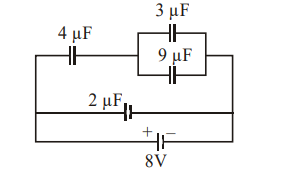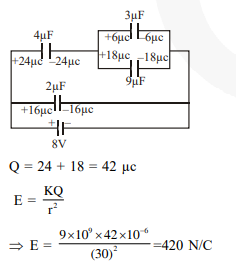Deepak Scored 45->99%ile with Bounce Back Crack Course. You can do it too!

# A combination of capacitors is set up as shown in the figure.

Question:

A combination of capacitors is set up as shown in the figure. The magnitude of the electric field, due to a point charge $\mathrm{Q}$ (having a charge equal to the sum of the charges on the $4 \mu \mathrm{F}$ and $9 \mu \mathrm{F}$ capacitors), at a point $30 \mathrm{~m}$ from it , would equal:1. $480 \mathrm{~N} / \mathrm{C}$

2. $240 \mathrm{~N} / \mathrm{C}$

3. $360 \mathrm{~N} / \mathrm{C}$

4.  $420 \mathrm{~N} / \mathrm{C}$

Correct Option: , 4

Solution: